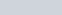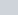# Work done : basic meaning, core concepts, work done by force, unit, factors affecting work done

• 18
•
•
•
•
•
•
•
18
Shares

## Introduction

In our daily life we use the word “work” when we do something like we push a car for some distance , we pull our bag or luggage  ,we lift something from ground floor to upper floors and in so many things we use the word work.

Also when we do work we feel getting tired and We think to have less energy or or energy is used in doing work this implies that work and energy are somehow related therefore this concept of work and energy were are familiar with our daily life experiences.

We use the word Work a lot of times in our life and in Physics The Concept of Work and energy is very useful , this concept of work Done that we are going to study today will be used in Electromagnetism and in understanding the concept of Potential Energy , the work done by springs , and in the theorems of Energy Conservation and lot more things the same concept will be used to define power. So the Concept of Work is of its own importance.

## Defining Work

Now from the perspective of a physics student or physicist we should define the work so that we can say when a work is done or not.

So in physics work is defined as

“A force performs work if point of action of force gets displaced with time”

Also  work is something which changes kinetic energy of body . You have to do net   work to change kinetic energy of body.

Point of Action and line of action of forceWork depends on frame of reference as displacement depends on frame of reference.

When we do work there is consumption of energy , hence work is somehow related to energy.

Hence work is a scalar quantity and having SI unit Joule

Mathematical Definition of Work

mathematical work is defined seperately for constant forces and variable forces.

#### Work done by constant force

In physics a constant force is a force whose magnitude and direction doesn’t changes means both magnitude and direction are constant.

For constant forces MathematicallySI Unit = Joule (J)

CGS Unit = erg , 1 J = 10⁷ erg

Note: From the above definition one may argue that if we take a bag or something in our head and stand at a position keeping the displacement of bag zero , our energy is being consumed so work should be done.

But In physics we say that work is zero as defined , so this means that our definition of Work Need not to be physically satisfied in every Situation, it’s a term defined by us.

Also in Physics Wherever the term Work Comes we think it be our defined term.

##### Why work is defined as dot product of force and displacement ?

Consider the ideal case , a toy car is moving with uniform velocity with  in x direction linearly and you are pushing it downwards (perpendicular to direction of motion) i.e angle b/w Force and Displacement is 90 degrees , and there are no frictional forces present. Then the speed of car doesn’t change i.e it’s kinetic energy doesn’t change and here we can see dot product of force and displacement is minimum i.e zero ,Now if we slightly change the angle of applied force then the speed of car will change if it’s towards direction  of motion then speed increases and if it’s opposite to Direction of motion then speed decreases and if we make the direction of applied force towards displacement i.e. angle b/w force and displacement = 0 degree  or 180 degrees then the speed of car will change by max amount i.e change in kinetic energy is maximum and here the dot product of force and displacement also comes out to be maximum.

So. Considering such examples we can assign work a meaning that work is done then speed of objects changes ,kinetic energy changes and it is appropriate from observation that the dot product of Force and Displacement is suitable to define work.

#### Work done by Variable force

Here  Work is defined for a force which Varies with PositionAnd for the evaluation of work done by such variable forces ,

The path on which the particle experiences this variable force is divided into very small  parts  such that the whole path is divided into infinite parts  we say this infinitesimal parts.Now in an infinitesimal small displacement(denote very small displacement )

In each such infinitesimal small displacement the change , variation of force will be very small and We can assume the force to be constant in an infinitesimal part

Now in an infinitesimal part of displacement dS the work de will be –Here the Integration Performs the following things

• It evaluates the work in each infinitesimal ds displacements

• It add all thes works and gives us the Final Work.

## Factors Affecting Work Done by a force

As we defined the work done by constant force and variable force both includes the dot product between Force vector and displacement vector.

So the work done by any force depends on following things

•  Force

• displacement

• angle between Force vector and displacement vector since the dot product is in the expression of work.